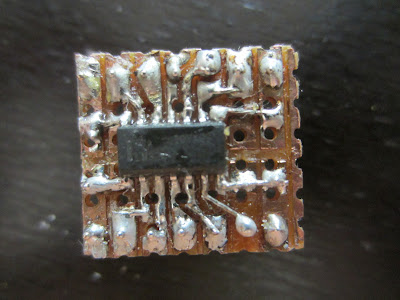## Tuesday, 26 February 2013

### Measuring inductances using an arduino

I just read this excellent article about measuring inductances:

http://reibot.org/2011/07/19/measuring-inductance/

The technique consists in measuring the resonance frequency of a LC circuit. Since this frequency is:

f = 1.0/(2.0*M_PI*sqrt(L*C))

By measuring f with the arduino and knowing C you can easily get L.

I modified the code so its much more precise, the original code is using 'pulse' to read half an oscillation. My code instead measures one whole oscillation by using an AVR's hw feature called Input Compare, this feaure starts and stops a timer that runs at 16Mhz.

Enjoy!

```//measuring inductance using the higher precision input compare
//based on http://reibot.org/2011/07/19/measuring-inductance/
//capture Flag
volatile uint8_t Flag;
void InitTimer1(void)
{
cli();
//Set Initial Timer value
TCCR1A = 0;
TCCR1B = 0;
// Input Capture Noise Canceller
TCCR1B |= (1 << ICNC1);
//First capture on rising edge
TCCR1B |= (1 << ICES1);
//Start timer without prescaller
TCCR1B &= ~ ((1 << CS12) | (1 << CS11) | (1 << CS10));
TCCR1B |= (1 << CS10);
//Enable input capture and overflow interrupts
TIMSK1 = 0;
TIMSK1 |= (1 << ICIE1) | (1 << TOIE1);
Flag = 0;
sei();
}
volatile uint16_t Capt;
volatile uint16_t T1Ovs2;
//capture ISR
ISR(TIMER1_CAPT_vect)
{
if (Flag == 0)
{
//reset overflows
TCNT1 = 0;
T1Ovs2 = 0;
}
else if (Flag == 1)
{
Capt = ICR1;
//disable capture and overflow interrupts
TIMSK1 &= ~ ((1 << ICIE1) | (1 << TOIE1));
//clear pending interrupts
TIFR1 = (1 << ICF1) | (1 << TOV1);
}
//increment Flag
Flag++;
}
//Overflow ISR
ISR(TIMER1_OVF_vect)
{
T1Ovs2++; //increment overflow counter
}
//13 is the input to the circuit (connects to 150ohm resistor)
//8 is the comparator/op-amp output.
//reibot.org for guide
double pulse, freq, inductance;
//insert capacitance here. Currently using 2uF
double capacitance = 2E-6;
void setup()
{
Serial.begin(115200);
pinMode(8, INPUT);
digitalWrite(8, LOW);
pinMode(13, OUTPUT);
Serial.println("Why hello!");
delay(200);
}
void loop()
{
digitalWrite(13, HIGH);
delay(10); //give some time to charge inductor.
digitalWrite(13, LOW);
delayMicroseconds(15); //make sure resonance is measured
InitTimer1();
delay(100); // wait for ICR to read once cycle
pulse = (T1Ovs2 * 65536 + Capt) / 16.0;
if (pulse > 0.1)
{
freq = 1.E6 / pulse;
inductance = 1. / (capacitance * freq * freq * 4. * 3.14159 * 3.14159);
inductance *= 1E6;
Serial.print("High for uS:");
Serial.print(pulse);
Serial.print("\tfrequency Hz:");
Serial.print(freq);
Serial.print("\tinductance uH:");
Serial.println(inductance);
delay(20);
}
}```

## Sunday, 3 February 2013

### SMD if there is a will there is a way

I couldn't wait for my breakout boards to arrive! I hope this encourages others to do some smd soldering... I used some rosin soldering flux from radio shack and a regular solder.SMD breakout board